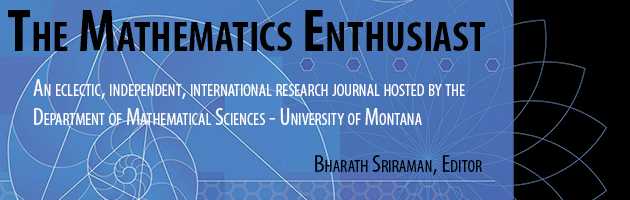•
•11

3

#### Abstract

The Cantor-Schroeder-Bernstein theorem states that any two sets that have injections into each other have the same cardinality, i.e. there is a bijection between them. Another way to phrase this is if two sets A;B have monomorphisms from A to B and B to A, then they are isomorphic in the setting of sets. One naturally wonders if this may be extended to other commonly studied systems of sets with structure and functions which preserve that structure. Given two objects with injective structure preserving maps between them are the structures of these objects the same? In other words, would these two objects be isomorphic in their respective setting? We see that in vector spaces, which are determined completely by their bases sets, this is true. However, when the objects are graphs, groups, rings or topological spaces, one may find counterexamples to such an extension. This is interesting, as it contradicts the naive intuition that two objects which are "subobjects" of each other must be the same. In this paper we provide some of these counterexamples.

475

484

COinS